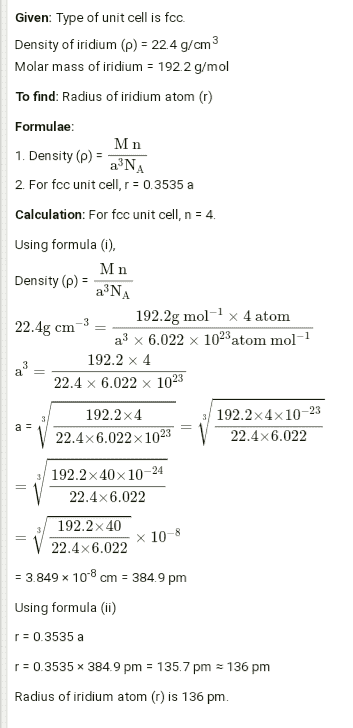# The density of iridium is 22.4 g/cm3 . The unit cell of iridium is fcc. Calculate the radius of iridium atom. Molar mass of iridium is 192.2 g/mol.

The density of iridium is 22.4 g/cm3  The unit cell of iridium is fcc. Calculate the radius of iridium atom. Molar mass of iridium is 192.2 g/mol.